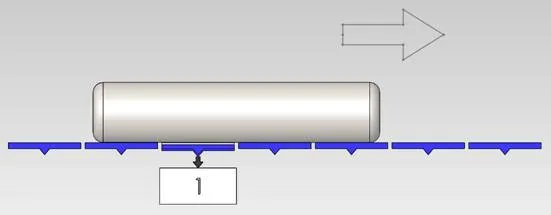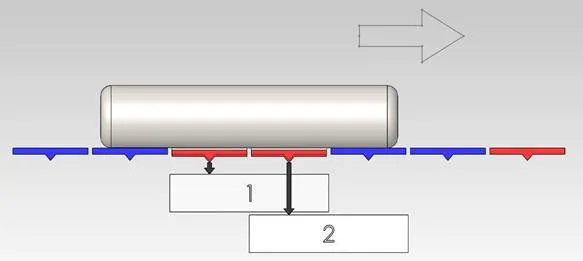# Compac

Estimating the Weight of a Moving Article Across Multiple Weigh Points## Problem Specification

The problem stated here aimed at designing a mathematical model for accurately estimating weight of a moving object from noisy and heavily biased signals involving both known and unknown sources of data contamination.

### Goal

To design a mathematical model for accurately estimating an article’s weight when it is supported by multiple points that are weighed at different times, on a rapidly moving conveyor system.

The main challenge arising from the proposed weighing system design is related to the fact that as different (contact) points where an article is supported, on the conveyor, pass over a load cell, the load cell is required to flex in order to get a reading of the weight. It is this flexing of the load cell, which will potentially redistribute some weight to other supporting points during the weighing process.

Also, as linear speed across the weighing platform is increased, the time available for ‘transition onto load cell’ vibrations to dissipate is reduced.

In order to double the time period for weighing on the load cell, we have a system where we can weigh two consecutive weigh points at the same time on separate load cells. The data from this system and a single-load cell system will be provided for comparison.

### Desired Outcomes

• Propose a model for estimating article weight from the provided datasets, which is robust to the article position and weight distribution on the weigh points.
• Determine the theoretical weighing accuracy expected at a range of speeds, including the maximum linear speed for which we can achieve sufficient weighing accuracy (will be specified).
• Compare the accuracy of the two-load cell system with the single-load cell system.

### Data

• Two sets of load cell data available as the weigh points pass over the load cell(s) carrying articles under different parameters:
1. A single load cell that weighs each weigh point one after another.
2. Two load cells creating alternating weigh points to allow longer weighing time.
• Static weights obtained from the above two system settings.
• Physical length of the different weigh sections (load cell plates).
• Weight of the weigh points themselves.